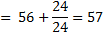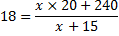## Average For SBI PO set 1

1) Average score of Rahul, Manish and Suresh is 63. Rahul’s score is 15 less than Ajay and 10 more than Manish. If Ajay scored 30 marks more than the average score of Rahul, Manish and Suresh, what is the sum of Manish’s and Suresh’s scores?

(a) 120

(b) 111

(c) 117

(d) Cannot be determined

(e) None of these

(b) 111

Explanation:

Ajay’s score = 63 + 30 = 93

Rahul’s score = 93 – 15 = 78

Sum of Manish’s and Suresh’s scores

= 3 x 63 – 78 = 189 – 78 = 111

2) The sum of 8 consecutive odd numbers is 656. Also average of four consecutive even numbers is 87. What is the sum of the smallest odd number and second largest even number?

(a) 165

(b) 175

(c) 163

(d) Cannot be determined

(e) None of these

(c) 163

Explanation:

Average of 8 consecutive odd numbers = (656/8) 82

Fourth number = 82 – 1 = 81

First number = 75

Average of 4 even numbers = 87

Second even number = 87 – 1 =86

Second largest even number = 88

Required sum = 75 + 88 = 163

3) The average marks in English subject of a class of 24 students are 56. If the marks of three students were misread as 44, 45 and 61 of the actual marks 48, 59 and 67 respectively, then what would be the correct average?

(a) 56

(b) 55

(c) 57.5

(d) 58.5

(e) None of these

(e) None of these

Explanation:

Difference = 48 + 59 + 67 44 – 45 – 61 =24

Correct average4) The average age of some males and 15 females is 18 years. The sum of the ages of 15 females is 240 years and average age of males is 20 years. Find the number of males.

(a) 8

(b) 7

(c) 10

(d) 15

(e) None of these

(d) 15

Explanation:

Let the number of males be x.18x + 270 = 20x + 240

20x – 18x = 270 – 240

2x = 30

x=30/2=15

5) The sum of the present ages of P and Q is 25 years more than the age of R. The present age of is 5 years more than that of R. Find the present age of P.

(a) 20 years

(b) 25 years

(c) 21 years

(d) 22 years

(e) None of these

(a) 20 years

Explanation:

P + Q = R + 25 … (i)

Q = R + 5 …….. (ii)

Subtracting equation (ii) from equation (i),

P+Q-Q=R+ 25 – R – 5

P = 20 years

6) The average of four consecutive even numbers P, Q, R and S respectively (in increasing order) is 51. What is the product of P and R?

(a) 2592

(b) 2400

(c) 2600

(d) 2808

(e) None of these

(e) None of these

Explanation:

x+x+2+x+4+x+6

= 4 x 51

4x + 12 = 204

4x = 204 – 12 = 192

x =  192/4  = 48 = P

R = 48 + 4 = 52

P x R = 48 x 52 = 2496

7) The average height of 27 per-sons was recorded as 162 cm. If the height of Shreya was deleted from the observation, the average height reduced by I cm. What was Shreya’s height?

(a) 184 cm.

(b) 226 cm.

(c) 179 cm.

(d) 186 cm.

(e) None of these

(e) None of these

Explanation:

Tricky approach

Height of Shreyas

= 162+26

= 188 cm

8) The average of the 9 consecutive positive integers is 63. The product of the largest and smallest integer is

(a) 3935

(b) 3953

(c) 3853

(d) 3835

(e) 3635

(b) 3953

Explanation:

Average of 9 consecutive positive integers = 63

Fifth number = 63

Smallest number = 59

Largest number = 67

Required product 47= 59 x 67 = 3953

9) The present age of Romila is one fourth of that of her father. After 6 years the father’s age will be twice the age of Kapil. If Kapil celebrated fifth birth day 8 years ago, what is Romila’s Present age?

(a) 7 years

(b) 7.5 years

(c) 8 years

(d) 8.5 years

(e) None of these

(b) 7.5 years

Explanation:

Kapil’s present age  = 12 years

After 6 years, Kapil’s age  = 18 years

Father’s present age =36-6

= 30 years

Romila’s present age

(¼)  ×30=7.5years

10) The average height of 16 students is 142 cm. If the height of the teacher is included, the average height increases by 1 cm. The height of the teacher is

(a) 156 cm

(b) 159 cm

(d) 157 cm

(c) 158 cm

(e) 159.5 cm